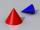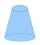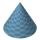# Rotary cone

The volume of the rotation of the cone is 472 cm3 and angle between the side of the cone and base angle is 70°. Calculate lateral surface area of this cone.

Result

S =  275.27 cm2

#### Solution:Leave us a comment of example and its solution (i.e. if it is still somewhat unclear...):

Showing 0 comments:Be the first to comment!#### To solve this example are needed these knowledge from mathematics:

See also our right triangle calculator. Most natural application of trigonometry and trigonometric functions is a calculation of the triangles. Common and less common calculations of different types of triangles offers our triangle calculator. Word trigonometry comes from Greek and literally means triangle calculation.

## Next similar examples:

1. Rotary coneRotary cone whose height is equal to the circumference of the base, has a volume 229 cm3. Calculate the radius of the base circle and height of the cone.
2. Cut and coneCalculate the volume of the rotation cone which lateral surface is circle arc with radius 15 cm and central angle 63 degrees.
3. RotationRight triangle with legs 14 cm and 20 cm rotate around longer leg. Calculate the volume and surface area of the formed cone.
4. Truncated coneCalculate the height of the rotating truncated cone with volume V = 1115 cm3 and a base radii r1 = 7.9 cm and r2 = 9.7 cm.
5. ConeIf the segment of the line y = -3x +4 that lies in quadrant I is rotated about the y-axis, a cone is formed. What is the volume of the cone?
6. ConeCalculate volume and surface area of ​​the cone with diameter of the base d = 15 cm and side of cone with the base has angle 52°.
7. Cone A2VSurface of cone in the plane is a circular arc with central angle of 126° and area 415 cm2. Calculate the volume of a cone.
8. SandpileAuto sprinkled with sand to an approximately conical shape. Workers wanted to determine the volume (amount of sand) and therefore measure the circumference of the base and the length of both sides of the cone (over the top). What is the volume of the san
9. ConeCircular cone with height h = 29 dm and base radius r = 3 dm slice plane parallel to the base. Calculate distance of the cone vertex from this plane, if solids have the same volume.
10. 2x coneCircular cone height 84 cm was cut plane parallel with base. Volume of these two small cones is the same. Calculate the height of the smaller cone.
11. Axial sectionAxial section of the cone is an equilateral triangle with area 208 dm2. Calculate the volume of the cone.
12. Cone and the ratioRotational cone has a height 23 cm and the ratio of the base surface to lateral surface is 7: 9. Calculate a surface of the base and the lateral surface.
13. Angle of deviationThe surface of the rotating cone is 30 cm2 (with circle base), its surface area is 20 cm2. Calculate the deviation of the side of this cone from the plane of the base.
14. Rotating cone IICalculate area of surface of rotating cone with base radius r=19 cm and height h=9 cm.
15. The coneThe lateral surface area of the cone is 4 cm2, the area of the base of the cone is 2 cm2. Determine the angle in degrees (deviation) of the cone sine and the cone base plane. (Cone side is the segment joining the vertex cone with any point of the base ci
16. TowerHow many m2 of copper plate should be to replace roof of the tower conical shape with diameter 24 m and the angle at the vertex of the axial section is 144°?
17. CanopyMr Peter has metal roof cone shape with a height of 127 cm and radius 130 cm over well. He needs paint the roof with anticorrosion. How many kg of color must he buy if the manufacturer specifies the consumption of 1 kg to 3.3 m2?